# Nor Flash Forum Discussions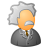Level 4
Level 4

# For S25FL128S, some questions about SDR AC Characteristics

Hi,

I am using the flash chip of CYPRESS,S25FL128SAGNFI000, but I have some questions that I don't understand  about the SDR AC Characteristics.

There is a question that I do not understand most.

At the datasheet of the chip, the 5.4 SDR AC Characteristics says that  ( tV Clock Low to Output Valid  can be 7.65 ns max ).(my stuation is note3 : 7.65 ns)

Here is the question, what is the most important influence factor to the time of tV?

In other words, when can the tV be the max value of 7.65 ns ? and when can the tV be the less value than 7.65 ns?

(In my design, the value of tV affect the reading timing sequence, so I want to make sure the tV can be controlled, otherwise my design will be influenced...)

So, wouly you help me to figure out this question?

Thank you very much!!!

1 SolutionModeratorModerator# Re: For S25FL128S, some questions about SDR AC Characteristics

Hi,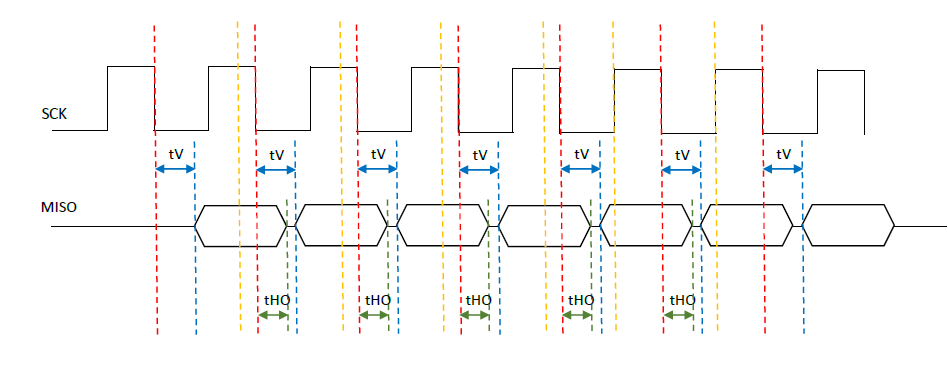Please refer the above diagram to understand about how tV parameter is defined from the flash point of view. Flash device will give data out during a read operation tV time after clock falling edge and it will be available on the SPI bus tHO time after the next clock falling edge. Data valid window to sample the data correctly by MCU will be as below.

Data valid window = Clock period (tPSCK) – tV(max) +tHO

Based on the available data valid window, MCU should decide when to sample the data while reading from flash. So, the maximum frequency with which a read operation can be performed depend upon the available data valid window and data setup time and data hold time requirements of MCU.

• Frequency of operation (fSCK) = 100 MHz
• Clock period (tPSCK) = 10ns
• tV (max) = 8ns
• tHO (output hold time of flash device) = 2ns

Data valid window = tPSCK – tV + tHO = 10 – 8 + 2 = 4 ns.

Let us consider the setup time and hold time requirements of MCU as below.

• Input setup time of MCU = 2 ns
• Input hold time of MCU = 2 ns
• Minimum data valid window required = 4ns

Then, it is possible to sample the data along with the falling edge of same SPI clock by MCU. See red line in the above diagram.

If the setup time and hold times of MCU are as below,

• Input setup time of MCU = 5 ns
• Input hold time of MCU = 2 ns
• Minimum data valid window required = 7ns

Since the data valid window available is only 4 ns, MCU won’t be able to sample output data correctly in this case. SPI clock frequency should be reduced to make the data valid window wider, such that the setup time and hold time of MCU are met.

Users can take below actions to make the data valid window wide, if the setup time and hold time requirements of MCU are not met.

1. Reduce CL to reduce tV value.
2. Reduce SPI clock frequency.

In the 'bad' case that you mentioned, you may need to reduce the SPI clock frequency to sample output data from flash at the rising edge of the clock.

Thanks and Regards,

Sudheesh

4 RepliesModeratorModerator# Re: For S25FL128S, some questions about SDR AC Characteristics

Hi,

tV is not a user controllable parameter. Could you please clarify, why you need to control tV parameter?

Thanks and Regards,
SudheeshLevel 4
Level 4

# Re: For S25FL128S, some questions about SDR AC Characteristics

Hi,

I am so sorry that I didn't make it clearly to understand...

I am using the FPGA to control the flash chip, and the flash SCK is 100MHz, the system clk of my FPGA is 200MHz.

For the FPGA, there is a important parameter that I can not control idealy, it is the signal delay in FPGA.

Such as if there is a signal of A coming into the pin of FPGA, but the signal A has to go through the FPGA to be detected, so there is the delay between the signal A coming into the pin and the signal A be detected.

For my design, the delay is about 5 ns.(it is not certain but nearly 5 ns, may be 6 ns or 4ns).

Here are the situations.(take the RDSR1 command as example)

Situation 1: Ideal case

In this case, it is same as the datasheet of the flash chip.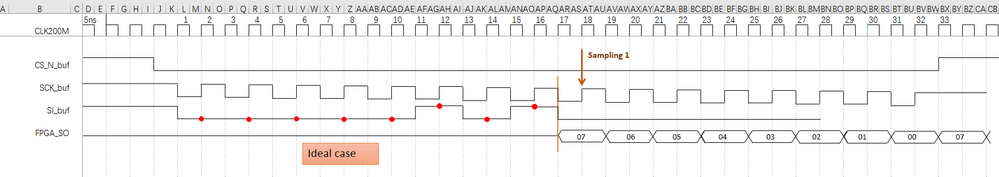As the figure, when the RDSR1 command is sent to the flash, the value comes out as the falling edge of SCK, as the brown line in the figure.

And in my design, I read the first SR1_value form the the Sampling1 (SCK rising edge).

And the design is OK.

(But this is ideal case, not the real situation.)

Situation 2 : Real case(Good)

In this case, the tV and the delay in FPGA are contained into the design.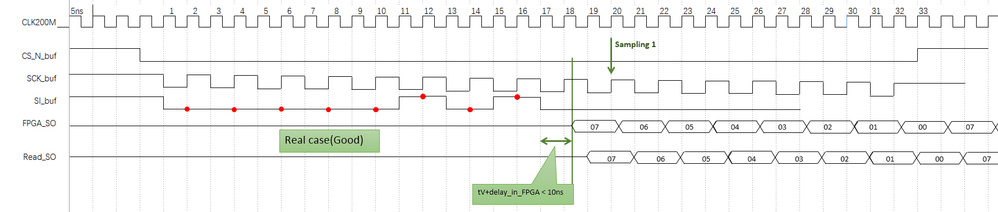In my design, I use the 200MCLK to buffer the SO, and I postpone the Sampling timing for 1 SCK to meet the correct value.

So If the tV(max in 7.65 ns) plus the delay in FPGA is less than 10ns, the design will be OK.

At this moment, the delayinFPGA is 5ns around, So if the tV is less than  5ns, the design wil be OK.

But if the tV is more than 5 ns, here is the situation 3.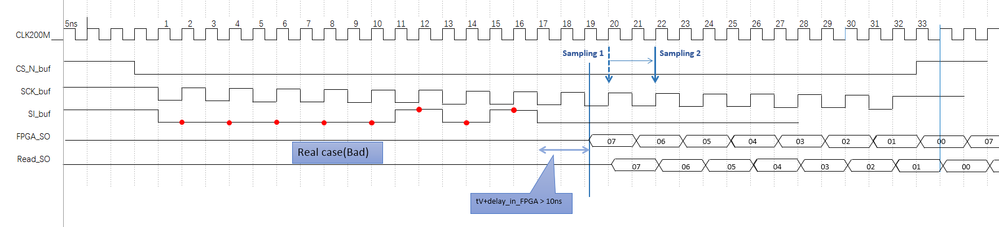In this case, if the tV is more than 5ns, which is the delayinFPGA plus the tV is more than 10ns, I must postpone the Sampling timing for 2 SCK to meet the correct value.

So here is the problem:

the tV is probably more than 5 ns and less than 5ns, which cause the Sampling timing delay for 1 or 2 SCK. but I must make sure the Sampling timing is certainly OK for every situation..

I just can not figure out what should I do..

Thank you very much!!ModeratorModerator# Re: For S25FL128S, some questions about SDR AC Characteristics

Hi,Please refer the above diagram to understand about how tV parameter is defined from the flash point of view. Flash device will give data out during a read operation tV time after clock falling edge and it will be available on the SPI bus tHO time after the next clock falling edge. Data valid window to sample the data correctly by MCU will be as below.

Data valid window = Clock period (tPSCK) – tV(max) +tHO

Based on the available data valid window, MCU should decide when to sample the data while reading from flash. So, the maximum frequency with which a read operation can be performed depend upon the available data valid window and data setup time and data hold time requirements of MCU.

• Frequency of operation (fSCK) = 100 MHz
• Clock period (tPSCK) = 10ns
• tV (max) = 8ns
• tHO (output hold time of flash device) = 2ns

Data valid window = tPSCK – tV + tHO = 10 – 8 + 2 = 4 ns.

Let us consider the setup time and hold time requirements of MCU as below.

• Input setup time of MCU = 2 ns
• Input hold time of MCU = 2 ns
• Minimum data valid window required = 4ns

Then, it is possible to sample the data along with the falling edge of same SPI clock by MCU. See red line in the above diagram.

If the setup time and hold times of MCU are as below,

• Input setup time of MCU = 5 ns
• Input hold time of MCU = 2 ns
• Minimum data valid window required = 7ns

Since the data valid window available is only 4 ns, MCU won’t be able to sample output data correctly in this case. SPI clock frequency should be reduced to make the data valid window wider, such that the setup time and hold time of MCU are met.

Users can take below actions to make the data valid window wide, if the setup time and hold time requirements of MCU are not met.

1. Reduce CL to reduce tV value.
2. Reduce SPI clock frequency.

In the 'bad' case that you mentioned, you may need to reduce the SPI clock frequency to sample output data from flash at the rising edge of the clock.

Thanks and Regards,

SudheeshLevel 4
Level 4

# Re: For S25FL128S, some questions about SDR AC Characteristics